Next: 14.3 Inverse Functions Up: 14. The Inverse Function Previous: 14.1 The Intermediate Value   Index

# 14.2 Applications

14.6   Example. We know that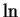is continuous on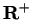, and that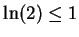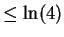.(Cf equation (5.78).) It follows that there is a number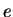in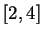such that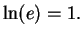14.7   Example. Two points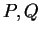on a sphere are called antipodal points if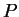and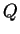are opposite ends of the same diameter of the sphere. We will consider the surface of the earth to be a sphere of radius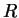. At any fixed time, let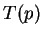denote the temperature of the earth at the point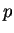on the surface of the earth. (More precisely, letbe the number such that the temperature atis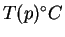). We will show that there are two antipodal pointson the surface of the earth such that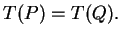In fact, we will show that there are two antipodal points on the equator with the same temperature. We first introduce a coordinate system so that the center of the earth is at the origin, and the plane of the equator is the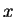-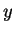plane, and the point on the equator passing through the Greenwich meridian is the point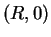. Then the points on the equator are the points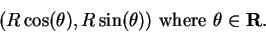Define a function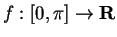by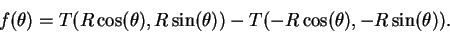Thus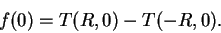We suppose that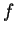is a continuous function on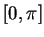. If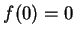then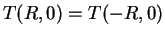, soand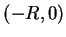are a pair of antipodal points with the same temperature. Now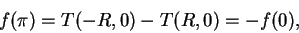so if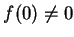then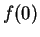and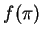have opposite signs. Hence by the intermediate value property, there is a number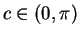such that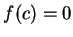, i.e.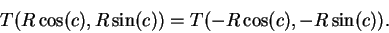Then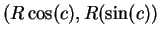and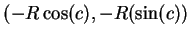are a pair of antipodal points with the same temperature.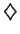14.8   Example. Let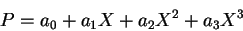where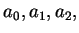and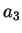are real numbers, and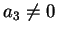. Then there exists some number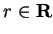such that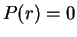.

Proof:     I will suppose that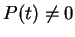for all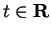and derive a contradiction. Let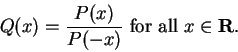Since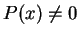for all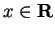,is continuous on R. We know that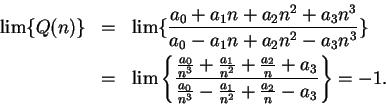Hence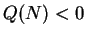for some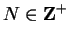. Then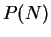and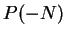have opposite signs, so by the intermediate value property there is a number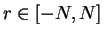such that. This contradicts our assumption thatfor all14.9   Exercise. A Let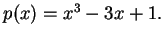Show that there are at least three different numbers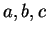such that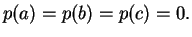14.10   Exercise. A Three wires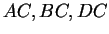are joined at a common point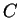.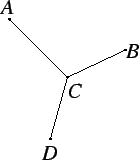Let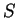be the Y-shaped figure formed by the three wires. Prove that at any time there are two points inwith the same temperature.

14.11   Exercise. A Six wires are joined to form the figure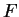shown in the diagram.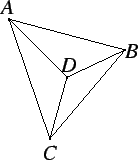Show that at any time there are three points inthat have the same temperature. To simplify the problem, you may assume that the temperatures at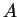,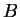,, and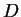are all distinct.Next: 14.3 Inverse Functions Up: 14. The Inverse Function Previous: 14.1 The Intermediate Value   Index
Ray Mayer 2007-09-07